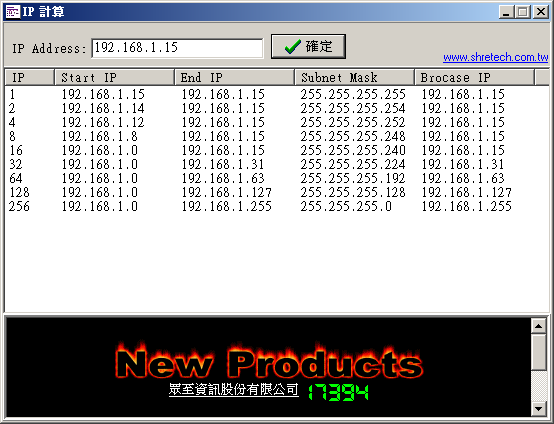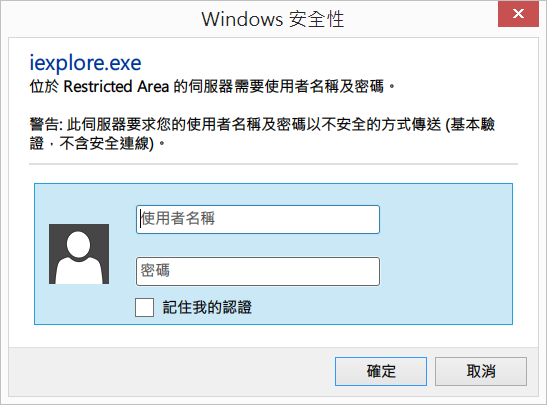## Archive for ‘ 十二月, 2004 ’

### Everything is Object in JavaScript

```alert (typeof('abc'));
var ary = [123, "abc"];

### Create Objects in JavaScript

```var main = new Object;     // 建立新物件
main.x = 123; // 设定物件成员变量(属性)之一
main["y"] = "XYZ"; // 设定物件成员变量(属性)之二

### List All Members in an Object

```function listMember(main) {
var s = "";
for( key in main )  // 使用 in 运算子列举所有成员
s += key + ": " + main[key] + "\n";
return s;
}```

```var ary = [123, "abc"];

### Construct Object with Initial Value

```function Array2DVar(x,y) {  // 定义二维阵列原型
this.length = x;
this.x = x;  // x 维度长度
this.y = y;  // y 维度长度
for(var i = 0; i < this.length; i++)  // 初始各元素值为 null
this[i] = new Array(y);  // this 代表物件本身
}

var a2dv = new Array2DVar(10, 10);  // 建立新的 10*10 的二维阵列
a2dv = "ABC";  // 设定二维阵列元素值
a2dv = "XYZ";
a2dv = 1000;

### Initial Array Object

```a = new Array("abc", "xyz", 1000); // constructor 语法，或
a = ["abc", "xyz", 1000]; // 阵列标准语法```

```b = [ // 使用函式作为阵列元素
];

for (var i = 0; i < b.length ; i++)
b[i]();```

### Object as Association Array

```obj1 = {"a" : "Athens" , "b" : "Belgrade", "c" : "Cairo"};
obj2 = {
name: "Edward",
showName: function() { alert(this.name); }  // 使用函式作为物件属性
}
obj2.showName();  // 显示 Edward
obj2.age = 23;  // 属性可以动态加入```

### Object as Return Value

```function a () {
return [32, 17];
}
b = a();
function pixel () {
return {"x": 32, "y":17};
}
point = pixel ();
alert (point.x + "\n" + point.y);  // 或
alert (pixel().x + "\n" + pixel().y);```

### Delegation Function Object

```function doloop(begin, end, func) {  // 这个函式是个 iterator
for (var i = begin; i < end; i++) {
func(i);
}
}
function func1(i) {  // 印出 ** n **
document.writeln("** " + i + " **<br>");
}
doloop(1, 10, func1);  // 印出 10 行 ** n **
doloop(20, 29,
function(i) {  // 印出 10 行 ## n ##
document.writeln("## " + i + " ##<br>");
}
);```

### Object = Properties + Behaviors

```function Array2D(x,y){  // 以一维阵列模拟二维阵列的原型物件
this.length = x * y;  // 阵列总长
this.x = x;  // x 维度长度
this.y = y;  // y 维度长度
for(var i = 0; i < this.length; i++)  // 初始各元素值为 null
this[i] = null;
this.get = function(x,y){  // 成员函式：取得阵列第 [x,y]个元素值
return this[x*this.x + y];
}
this.set = function(x,y,value){  // 成员函式：设定阵列第 [x,y] 个元素值
this[x*this.x + y] = value;
}
}```

```var a2d = new Array2D(10, 10);  // 建立新的“二维”阵列

a2d.set(1, 3, "ABC");  // 设定“二维”阵列元素值
a2d.set(2, 6, "XYZ");
a2d.set(9, 9, 1000);

### Member Function Outside of Constructor

```function Array2D(x,y){  // 以一维阵列模拟二维阵列的原型物件
this.length = x * y;  // 阵列总长
this.x = x;  // x 维度长度
this.y = y;  // y 维度长度
for(var i = 0; i < this.length; i++)  // 初始各元素值为 null
this[i] = null;
this.get = Array2DGet;  // 用这种方式把成员函式挂进来
this.set = Array2DSet;
}

function Array2DGet(x,y){  // 成员函式：取得阵列第 [x,y] 个元素值
return this[x*this.x + y];
}

function Array2DSet(x,y,value){  // 成员函式：设定阵列第 [x,y] 个元素值
this[x*this.x + y] = value;
}```

### Dynamic Object Function

```function array_max(){  // 定义求取 Array 最大值之函式
var i, max = this;
for (i = 1; i < this.length; i++){
if (max < this[i])
max = this[i];
}
return max;
}
Array.prototype.max = array_max;  // 在 Array 原型中加入 max 函式```

```var x = new Array(1, 2, 3, 4, 5, 6);  // 透过 Array 建构子建立一阵列，想求取 x 中某一元素之最大值
var y = x.max( );  // 取得 x 之最大元素```

### Dynamic Mix in

```function main(){  // main 之建构子
// ...
this.imports =
function (object) {
if( typeof object == "object")
for( value in object )
this[value] = object[value];
}
// ...
}
obj = new main();
main.imports(new dynamic());  // 汇入 dynamic 物件之所有功能```

### IP 位址区段计算程式 *

• 名　　称: 计算IP(Stip)
• 档案大小: 526 KB
• 语言接口: 中英文版
• 版本性质: Freeware
• 释出日期: 2002/10/17
• 作业平台: Windows 9x/NT/2000/XP
• 原创网站: 众智资讯
• 档案下载:

• IP 数
• 开始的 IP(Network IP)
• 结束的 IP(Brocase IP)### .htaccess 的权限认证方式

“.htaccess 验证”让你不用写程式，就可以用最简单的方式做出“网页登入”的功能，限制哪些用户进到网页的某一层目录。

### 建立用户验证资料

#### 建立用户验证档

`htpasswd -c /path/to/.htusers UserName1`

`htpasswd /path/to/.htusers UserName2`

#### 建立群组资料档

`Group1:UserName1 UserName2`

### 验证登入

#### 针对用户:

```AuthName "Restricted Area"
AuthType Basic
AuthUserFile /path/to/.htusers

`require valid-user`

#### 针对群组:

```AuthName "Restricted Area"
AuthType Basic
AuthUserFile /path/to/.htusers
AuthGroupFile /path/to/.htgroups
require group Group1```### 问题排除

`AllowOverride All`

### Who Is Connected 1.6.1 - 让骇客现形

• 名　　称: Who Is Connected
• 版　　本: 1.6.1003.37
• 档案大小: 903.35 KB
• 语言接口: 英文版
• 版本性质: Freeware
• 作业平台: Windows 2000/XP
• 原创网站: Native Computer Systems
• 档案下载:

### [转载]Unicode 脱逸序列转换

```<META HTTP-EQUIV="Content-Type" CONTENT="text/html; charset=big5">
<script language=javascript>
<!--
function StringToUniCode(source) {
sret = "";
// Unicode 跳脱序列之格式为 \u00ff
for(var i=0; i < source.length; i++) {
var num = new Number( source.charCodeAt(i));
sret += "\\u" + num.toString(16);
}
return sret;
}
function convert(){
document.forms.content.result.value = StringToUniCode(document.forms.content.source.value);
}
//-->
</script>

<form name=content>

<input type=button value=转换 onclick="convert()">
</form>```